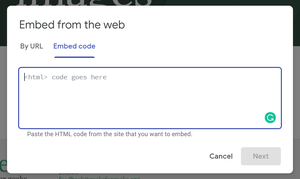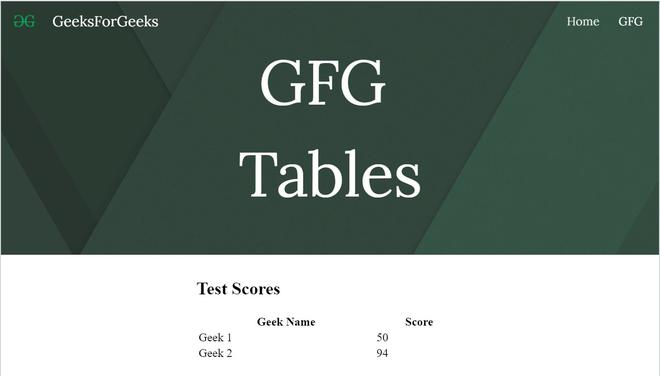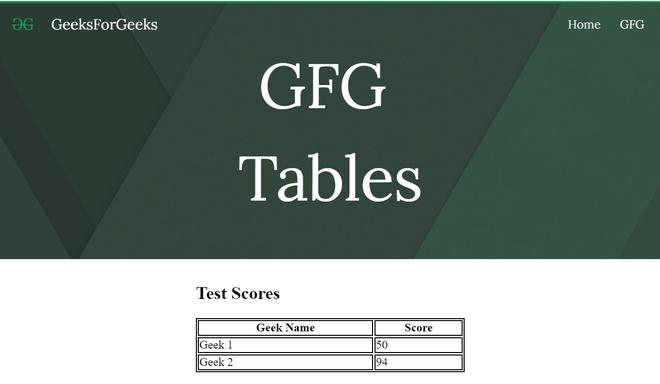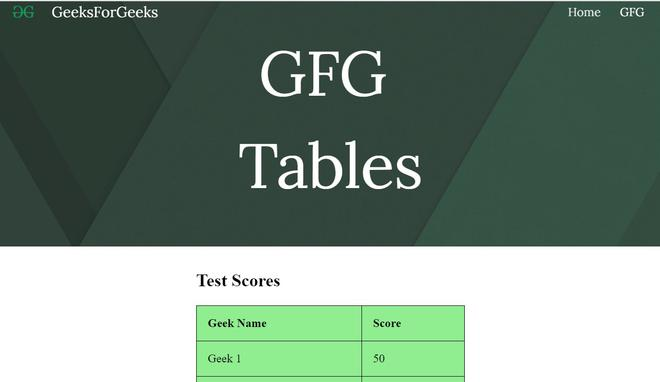Related Articles
How to embed tables in New Google Sites ?
• Last Updated : 10 Sep, 2020Sometimes, we have to display some data in a systematic manner, to do so we use tables. To embed the table in Google sites follow the steps:

Select embed option from the insert panel and then go to embed code division of the dialogue box appeared.Write your iframe code in the space provided.

• To insert a border-less table use the following code:

## HTML

 `<``body``>``    ``<``h2``>Test Scores``    ``<``table` `style``=``"width:100%"``>``        ``<``tr``>``            ``<``th``>Geek Name``            ``<``th``>Score``        ````        ``<``tr``>``            ``<``td``>Geek 1``            ``<``td``>50``        ````        ``<``tr``>``            ``<``td``>Geek 2`` ` `            ``<``td``>94``        ```` ` `    `````• To add a bordered table just use the following code:

## HTML

 `<``head``>``    ``<``style``>``        ``table,``        ``th,``        ``td {``            ``border: 1px solid black;``        ``}``    `````` ` `<``body``>``    ``<``h2``>Test Scores``    ``<``table` `style``=``"width:100%"``>``        ``<``tr``>``            ``<``th``>Geek Name``            ``<``th``>Score``        ````        ``<``tr``>``            ``<``td``>Geek 1``            ``<``td``>50``        ````        ``<``tr``>``            ``<``td``>Geek 2``            ``<``td``>94``        ````    `````• To add a table with some background color use the following code:

## HTML

 `<``head``>``    ``<``style``>``        ``table,``        ``th,``        ``td {``            ``border: 1px solid black;``            ``border-collapse: collapse;``        ``}`` ` `        ``th,``        ``td {``            ``padding: 15px;``            ``text-align: left;``            ``background-color: lightGreen;``        ``}``    `````` ` `<``body``>``    ``<``h2``>Test Scores``    ``<``table` `style``=``"width:100%"``>``        ``<``tr``>``            ``<``th``>Geek Name``            ``<``th``>Score``        ````        ``<``tr``>``            ``<``td``>Geek 1``            ``<``td``>50``        ````        ``<``tr``>``            ``<``td``>Geek 2``            ``<``td``>94``        ````    `````You can change the style and content of the table as per your requirement.

Attention reader! Don’t stop learning now. Get hold of all the important DSA concepts with the DSA Self Paced Course at a student-friendly price and become industry ready.

My Personal Notes arrow_drop_up